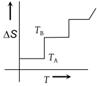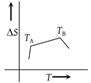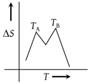Ozone is prepared by passing silent electric discharge through oxygen. In this reaction [AFMC 1998]

(1) Energy is given out

(2) Energy is absorbed

(3) Oxygen is loaded with energy

(4) Oxygen is dissociated into atoms

Concept Questions :-

Thermochemistry
High Yielding Test Series + Question Bank - NEET 2020

Difficulty Level:

Combustion of glucose takes place according to the equation, ${C}_{6}{H}_{12}{O}_{6}+6{O}_{2}\to 6C{O}_{2}+6{H}_{2}O$, $\Delta H=-72\text{\hspace{0.17em}}kcal$. How much energy will be required for the production of 1.6 g of glucose (Molecular mass of glucose = 180 g) [AFMC 1999]

(1) 0.064 kcal

(2) 0.64 kcal

(3) 6.4 kcal

(4) 64 kcal

Concept Questions :-

Enthalpy and It's Type
High Yielding Test Series + Question Bank - NEET 2020

Difficulty Level:

Which of the following compounds will absorb the maximum quantity of heat when dissolved in the same amount of water ? The heats of solution of these compounds at 25°C in kJ/mole of each solute is given in brackets [AMU (Engg.) 2000]

(1) $HN{O}_{3}\left(\Delta H=-33\right)$

(2) $KCl\left(\Delta H=+17.64\right)$

(3) $N{H}_{4}N{O}_{3}\text{\hspace{0.17em}}\left(\Delta H=+25.5\right)$

(4) $HCl\text{\hspace{0.17em}}\left(\Delta H=-74.1\right)$

Concept Questions :-

Thermochemistry
High Yielding Test Series + Question Bank - NEET 2020

Difficulty Level:

A system is changed from state A to state B by one path and from B to A another path. If E1 and E2 are the corresponding changes in internal energy, then [Pb. PMT 2001]

(1) ${E}_{1}+{E}_{2}=-ve$

(2) ${E}_{1}+{E}_{2}=+ve$

(3) ${E}_{1}+{E}_{2}=0$

(4) None of these

Concept Questions :-

Internal Energy and work done
High Yielding Test Series + Question Bank - NEET 2020

Difficulty Level:

If (i) $C+{O}_{2}\to C{O}_{2}$, (ii) $C+1/2{O}_{2}\to CO$, (iii) $CO+1/2{O}_{2}\to C{O}_{2}$, the heats of reaction are Q, –12, –10 respectively. Then Q = [Orissa JEE 2004]

(1) – 2

(2) 2

(3) – 22

(4) – 16

Concept Questions :-

Hess's Law
High Yielding Test Series + Question Bank - NEET 2020

Difficulty Level:

Adsorption of gases on solid surface is generally exothermic because [IIT JEE (Screening) 2004]

(1) Enthalpy is positive

(2) Entropy decreases

(3) Entropy increases

(4) Free energy increase

Concept Questions :-

Spontaneity and Entropy
High Yielding Test Series + Question Bank - NEET 2020

Difficulty Level:

Two mole of an ideal gas is expanded isothermally and reversibly from 1 litre ot 10 litre at 300 K. The enthalpy change (in kJ) for the process is [IIT JEE (Screening) 2004]

(1) 11.4 kJ

(2) –11.4 kJ

(3) 0 kJ

(4) 4.8 kJ

Concept Questions :-

CP and CV and Relation for Real Gas
High Yielding Test Series + Question Bank - NEET 2020

Difficulty Level:

When a gas undergoes adiabatic expansion, it gets cooled due to

1. Loss of kinetic energy

2. Fall in temperature

3. Decrease in velocity

4. Energy increase during doing work

Concept Questions :-

Thermodynamics' Properties and process
High Yielding Test Series + Question Bank - NEET 2020

Difficulty Level:

In an isobaric process, the ratio of heat supplied to the system (dQ) and work done by the system (dW) for diatomic gas is [AFMC 2002]

(1) 1 : 1

(2) 7 : 2

(3) 7 : 5

(4) 5 : 7

Concept Questions :-

CP and CV and Relation for Real Gas
High Yielding Test Series + Question Bank - NEET 2020

Difficulty Level:

If for a given substance melting point is TB and freezing point is TA, then correct variation shown by graph between entropy change and temperature is [DCE 2001]

(1)(2)(3)(4)Concept Questions :-

Spontaneity and Entropy